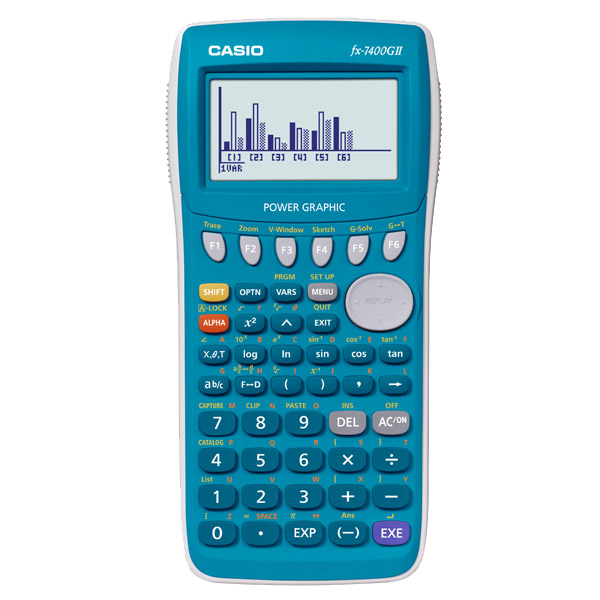# CASIO 7400GII Graphing Calculator

18,500.00

In stock

• High-definition display (64×128 dots)
• Inequality Graphing
• Polar Graph
• X= Graph
• Graph Solve Function (Root, Intersection)
• Sketch (Tangent)
• Bar Graph/Pie Chart
• Random Number Function
• Quotient, Remainder
• String Functions
• Unit Conversion
• Solve Calculations (EQUA mode)
• GCD/LCM
• 12 Types of Regression
• Complex Calculations
• Catalog Function
• Polynomial Function(EQUA mode)
• Simultaneous Functions (EQUA mode)
• Base-n Calculation
• Display Language Setting
• Data communication (requires optional 3-pin cable, FA-124 USB for connecting with PC)
SKU: b7077-1-1-1 Tags: ,

18,500.00

### Description

• Basic Statistics Standard statistics functions such as Mean, SUM, Standard Deviation, and Regression

• Basic Mathematical Functions Trigonometric, Exponential logarithmic, etc.

• Table Generation: You can create a numerical table based on that expression by registering a function expression. In addition, graph functions can be represented by graphs.

• Intermediate Statistics More statistical functions such as Paired-variable statistical calculation, Quartiles and List display functions for speedy and thorough learning .

• Base-n calculation Binary/Octal/Hexadecimal

• Graphing

• AAA-size Battery

• Differentiation

• Connection to PC

• Equation

• Integration

•Metric conversion function You can convert a number in one unit to a number in a different unit.

• Complex number calculation

• High Resolution We are choosing a model with higher definition in our non-programmable model and a higher definition model with Programmable model.

### Specification